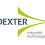### How is RTD's Error Compensated?

RTD's are used to measure temperature in plants. They are the most common Temperature sensors that are used in industries. Many of them claim that they are more accurate and reliable when it comes to temperature measurements. Guess what? they do have errors, but the thing is we can compensate for the errors.

We know, that Every lead wires have some resistance. Thus while taking RTD output ,this wire resistance can add to the measured RTD output and results in significant errors. These errors can be compensated by using the following methods.

2 wire configuration -

Lead wire resistance is a huge problem when it comes to 2 wire configuration. It cannot be compensated. In 2 wire RTD the connecting wires R1 and R2 has some resistance . So that there will  be higher resistance than the true output resistance. Thus it can be used only when accuracy is not our priority. Thus the only way to compensate in 2 wire configuration is to set a manual offset.

From the above image, we can see that R1 and R2 are lead resistance and they are being added to the total resistance which indeed is the output of RTD.

3 Wire Configuration -

It is the most commonly used configuration in industries . Error can be compensated only when each of the lead wires connecting the RTD has same resistance.

From the above image, R1,R2 and R3 are lead resistance and they are of same resistance. From the equation derived above it concludes that the error of 10 ohms is compensated.

If the lead wires used are not of same resistance this is how the equation will be

4 wire configuration -

Here lead wire compensation is done electrically not mathematically, by following ohms law. If each of the wire that connecting the rtd element is not same in 3 wire configuration error cannot be compensated. But in 4 wire even when each of the lead wire that connecting the RTD element is not the same ,wire resistance will be compensated

1.2.